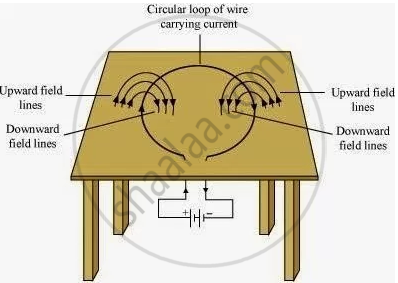Share

# Consider a Circular Loop of Wire Lying in the Plane of the Table. Let the Current Pass Through the Loop Clockwise. Apply the Right-hand Rule to Find Out the Direction of the Magnetic Field Inside and Outside the Loop - CBSE Class 10 - Science

ConceptMagnetic Field Due to a Current carrying Straight Conductor

#### Question

Consider a circular loop of wire lying in the plane of the table. Let the current pass through the loop clockwise. Apply the right-hand rule to find out the direction of the magnetic field inside and outside the loop

#### Solution

Inside the loop = Pierce inside the table
Outside the loop = Appear to emerge out from the table
For downward direction of current flowing in the circular loop, the direction of magnetic field lines will be as if they are emerging from the table outside the loop and merging in the table inside the loop. Similarly, for upward direction of current flowing in the circular loop, the direction of magnetic field lines will be as if they are emerging from the table outside the loop and merging in the table inside the loop, as shown in the given figure.Is there an error in this question or solution?

#### APPEARS IN

NCERT Solution for Science Textbook for Class 10 (2019 to Current)
Chapter 13: Magnetic Effects of Electric Current
Q: 1 | Page no. 229

#### Video TutorialsVIEW ALL 

Solution Consider a Circular Loop of Wire Lying in the Plane of the Table. Let the Current Pass Through the Loop Clockwise. Apply the Right-hand Rule to Find Out the Direction of the Magnetic Field Inside and Outside the Loop Concept: Magnetic Field Due to a Current carrying Straight Conductor.
S